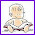### Concept of average

I felt like giving away something again to help all of you who need math lessons... This one is taken from Math Mammoth Division 2 book and is a lesson on the concept of average... meant for initial teaching for 4th-5th graders who have mastered long division.

I've written it quite recently, and in fact improved it just last night by adding the bar graph problems into it. (That seems to be the "way of life" with these books: I constantly keep tweaking and improving them as I learn. Which means constant uploading of files here and there... keeping our bandwidth usage large...)

The lesson mainly uses word problems, BTW. Please post your comments below; I'm anxious to hear them!

Right click and choose save:
Average_from_Math_Mammoth_Division_2.pdfmathmom said…
I like your lesson. But the one thing I would suggest changing is in your "backward" example you use average speed. Average speed is a topic that is full of pitfalls and deserves its own careful presentation. By casually presenting an average speed question here, among a set of "simple average" problems, you may feed the common misunderstanding that if one is given a bunch of speeds for different parts of a trip, one can find the average speed by simply averaging those numbers. For this reason, I'd stay away from "average speed" examples until you are ready to cover that topic more comprehensively.Maria Miller said…
Thanks; I changed it a bit now.Anonymous said…

Here is a useful way to compute an average for some number lists. Let me use three of your problems.

20 32 27 37 24

Pick any number and make it your reference value. Let's take 20. Now find the differences between the other numbers and this value: +12 +7 +17 + 4. Sum these: 40
Divide by 5 => 8. Add 20 + 8 = 28

A better variant of this is to pick the median or a value close to it as the reference number, so that the differences are minimized and the running sum is easier to manage as positive and negative numbers partially cancel each other out.

So take 27. The differences here are: -7 +5 +10 –3 = 5 5 / 5 = 1 27 + 1 = 28.

If the student writes each difference above the number in the list, and includes 0 above the reference number, this makes it easier to remember to divide the sum of differences by the correct divisor, here 5.

78 87 69 86

Take 78. The differences are +9 -9 +8, and sum these = +8
Divide 8 / 4 = 2. 78 + 2 = 80.

.

234 178 250 198

Take 198. Our differences are +36 –20 +52 => 68 / 4 = 17 198 + 17 = 215

This is a nice "unconventional alternative" method for finding averages of short lists of 2- and 3-digit numbers with relatively small differences. The student doesn't need to write a column of numbers to sum, and in these examples s/he does not need to perform long division, which take more time and are prone to generating trivial arithmetic errors. At the same time, it reminds students that they don't have to "automatically" resort to a calculator for problems that would, if tackled conventionally, normally call for one.Maria Miller said…
I actually use exactly that method myself, if I find an average with mental math.

I think it is a good addition to some later lesson on average. It does require some knowledge of negative numbers though.

In this lesson, the calculations didn't even involve decimals or fractions. In reality that would be the norm.